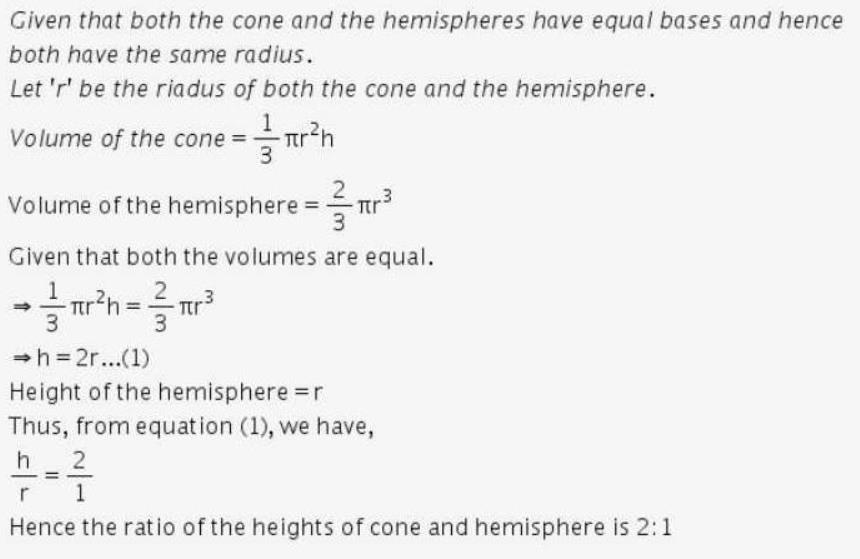Courses

# Practice Test: Surface Area & Volumes

## 25 Questions MCQ Test Mathematics (Maths) Class 10 | Practice Test: Surface Area & Volumes

Description
This mock test of Practice Test: Surface Area & Volumes for Class 10 helps you for every Class 10 entrance exam. This contains 25 Multiple Choice Questions for Class 10 Practice Test: Surface Area & Volumes (mcq) to study with solutions a complete question bank. The solved questions answers in this Practice Test: Surface Area & Volumes quiz give you a good mix of easy questions and tough questions. Class 10 students definitely take this Practice Test: Surface Area & Volumes exercise for a better result in the exam. You can find other Practice Test: Surface Area & Volumes extra questions, long questions & short questions for Class 10 on EduRev as well by searching above.
QUESTION: 1

### If the volume of a cube is 1728 cm3, the length of its edge is equal to

Solution:

Volume of the cube=a3=1728cm3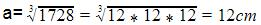QUESTION: 2

### Two cubes each of 10 cm edge are joined end to end. The surface area of the resulting cuboid is

Solution:

The length of the resulting cuboid=2 x 10 cm = 20 cm

Its width= 10 cm and its height = 10 cm

i.e. l=20cm, b=10cm, h=10 cm

∴The total surface area of the resulting cuboid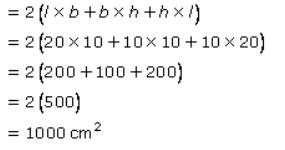QUESTION: 3

### A rectangular sheet of paper 44 cm × 18 cm is rolled along its length and a cylinder is formed. The volume of the cylinder so formed is equal to (Take π = 22/7)

Solution:
QUESTION: 4

If the radius and height of a cylinder are in the ratio 5 : 7 and its volume is 550 cm3, then its radius is equal to (Take π = 22/7)

Solution:

Let the radius = r = 5x and height = h = 7x

So, the volume of the cylinder = π r2 h

= 22/7 x (5x)2 x 7x

= 22/7 x 25x2 x 7x

= 22 x 25x (cube) (7 gets cancelled)

But it is given that volume = 550 cm cube

So, 22 x 25x (cube) = 550

x (cube) = 550 / 22 x 25

x(cube) = 1 ( as 22 x 25=550 and 550 is both in nm and dm)

x = 3

x = 1

so radius = 5x = 5 x 1 cm = 5cm

QUESTION: 5

If the curved surface area of a solid right circular cylinder of height h and radius r is one-third of its total surface area, then

Solution: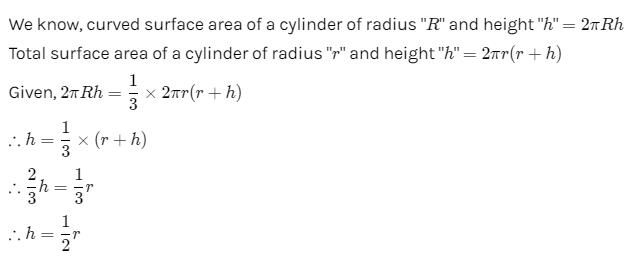QUESTION: 6

A hollow cylindrical pipe is 21 cm long. If its outer and inner diameters are 10 cm and 6 cm respectively, then the volume of the metal used in making the pipe is (Take π = 22/7)

Solution:

The height of the cylindrical pipe=21cm
volume of the metal used to make the Pipe=Π(R2-r2)h
=22/7×(102-62)
=1056cm3

QUESTION: 7

If the radius and slant height of a cone are in the ratio 4 : 7 and its curved surface area is 792 cm2, then its radius is (Take π = 22/7)

Solution:
QUESTION: 8

If the radius of the base and the height of a right circular cone are respectively 21 cm and 28 cm, then the curved surface area of the cone is (Take π = 22/7)

Solution:
QUESTION: 9

A conical tent with base-radius 7 m and height 24 m is made from 5 m wide canvas. The length of the canvas used is (Take π = 22/7)

Solution: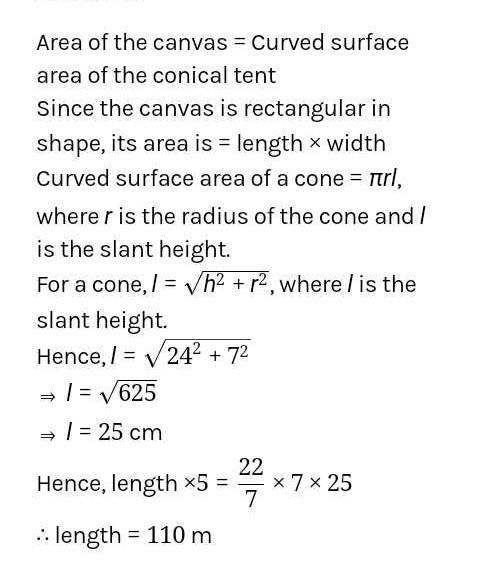QUESTION: 10

The total surface area of a solid hemisphere of radius 3·5 m is covered with canvas at the rate of Rs. 20 per m2. The total cost to cover the hemisphere is (Take π = 227)

Solution:

Total Surface Area of a hemisphere of radius 'r' =3πr2
Hence, total surface area of this hemisphere =3×22/7×3.5×3.5=115.5sqm
∴ cost of covering the hemisphere with canvas at the rate of Rs. 20 per m2=115.5×20=Rs2310

QUESTION: 11

If the volume of a vessel in the form of a right circular cylinder is 448 cm3 and its height is 7 cm, then the curved surface area of the cylinder is

Solution:

Volume of a Cylinder of Radius R and height h =πR2h
∴ volume of the given cylinder =π×R2×7=448πcm3

R2=64

R=8cm

Curved surface area of a cylinder of radius "R" and height "h" =2πRh

∴ curved surface area of the given cylinder =2×π×8×7=112π cm2

QUESTION: 12

If the curved surface area of a right circular cone is 12320 cm2 and its base-radius is 56 cm, then its height is (Takeπ = 22/7)

Solution:
QUESTION: 13

If a solid metallic sphere of radius 8 cm is melted and recasted into n spherical solid balls of radius 1 cm, then n =

Solution: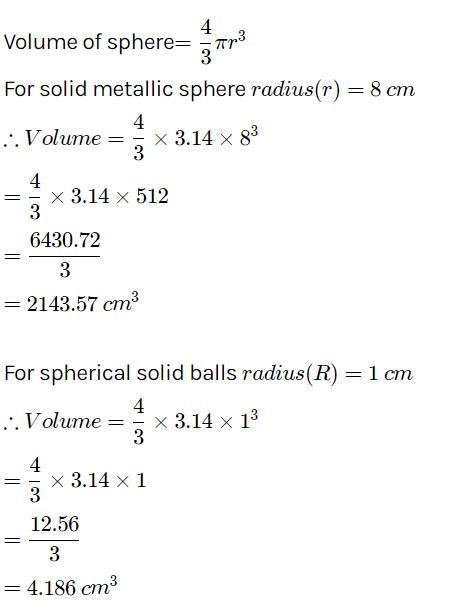In order to find the number of balls carved from larger sphere we need to divide the larger ball to small ball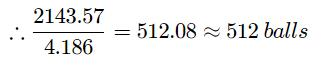QUESTION: 14

If the diameter of a metallic sphere is 6 cm, it is melted and a wire of diameter 0·2 cm is drawn, then the length of the wire made shall be

Solution:

We have
Diameter of metallic sphere =6cm
Also we have
Diameter of cross-section of cylindrical wire=0.2cm
∴ Radius of cross-section fo cylindrical wire=0.1cm
Let the length of the wire be h cm
Since the metallic sphere is converted into a cylindrical shaped wire of length h cm
Volume of the metal used in wire= Volume of the sphere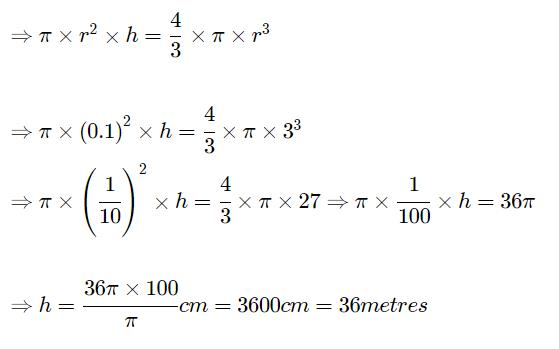QUESTION: 15

If n coins each of diameter 1·5 cm and thickness 0·2 cm are melted and a right circular cylinder of height 10cm and diameter 4·5 cm is made, then n =

Solution:
QUESTION: 16

A tent is in the form of a cylinder of diameter 8 m and height 2 m, surmounted by a cone of equal base and height 3 m. The canvas used for making the tent is equal to

Solution:
QUESTION: 17

A toy is in the form of a cone mounted on a hemisphere with same radius. The diameter of the base of the conical portion is 6 cm and its height is 4 cm. The surface area of the toy is

Solution:
QUESTION: 18

A frustum of a right circular cone is of height 16 cm with radii of its ends as 8 cm and 20 cm. The volume of the frustum is

Solution:
QUESTION: 19

A frustum of a right circular cone is of height 16 cm with radii of its ends as 8 cm and 20 cm has lateral surface area equal to

Solution:
QUESTION: 20

A solid metal cone with base-radius 12 cm and height 24 cm, is melted to form solid spherical balls, each of diameters 6 cm. The number of such balls made is

Solution:
QUESTION: 21

One cubic metre piece of copper is melted and recast into a square cross-section bar, 36 m long. An exact cube is cut off from this bar. If cubic metre of copper cost Rs. 108, then the cost of this cube is :

Solution:
QUESTION: 22

If the surface areas of two spheres are in the ratio 4 : 9, then the ratio of their volumes is :

Solution:
QUESTION: 23

In a shower 10 cm of rain fall. The volume of water that falls on 1.5 hectares of ground is :

Solution:
QUESTION: 24

The radius of base and the volume of a right circular cone are doubled. The ratio of the length of the larger cone to that of the smaller cone is :

Solution:
QUESTION: 25

A cone and a hemisphere have equal base diameter and equal volumes. The ratio of their heights is :

Solution: